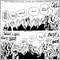# MT4:NormalizeDouble31000

NormalizeDouble(Close,0);

in order to remove the digits after the .

how to get the integer value that will not be modified

I am getting 2 for values like 1.6 while I need707

Use typecasting.

int nMy_Value = int(Close);

Check this:

https://www.mql5.com/en/docs/basis/types/castingDocumentation on MQL5: Language Basics / Data Types / Typecasting
• www.mql5.com
Language Basics / Data Types / Typecasting - Reference on algorithmic/automated trading language for MetaTrader 512468

maybe

int y = (int) NormalizeDouble(Close,0);19968

1. Do NOT use NormalizeDouble, EVER. For ANY Reason. It's a kludge, don't use it. It's use is always wrong
2. If you want the integer part of a double just cast it int y = int(d)
3. If you want the nearest multiple of a double, (round numbers,) compute it.
double MathNearest(  double v, double to){ return to * MathRound(v / to); }
double MathRoundDown(double v, double to){ return to * MathFloor(v / to); }
double MathRoundUp(  double v, double to){ return to * MathCeil( v / to); }
:
double   pip        = StringFind(_Symbol,"JPY") < 0 ? 0.01 : 0.0001;
int      pipDigits  = (int)MathLog10(pip/_Point);
int      slippage   = 3 * int(pip / _Point);

double market    = Bid;                             // 1.012345 122.012
double lower100  = MathRoundDown(market, 100*pip);  // 1.01200  122.00
double upper100  = MathRoundUp(  market, 100*pip);  // 1.01300  123.0031000

Snelle Moda:

Use typecasting.

int nMy_Value = int(Close);

Check this:

https://www.mql5.com/en/docs/basis/types/casting

whroeder1:

1. If you want the integer part of a double just cast it int y = int(d)
2. If you want the nearest multiple of a double, (round numbers,) compute it.